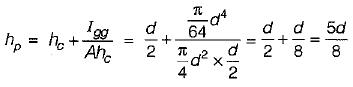Courses

# Test: Hydrostatic Force On Surface

## 10 Questions MCQ Test Topicwise Question Bank for GATE Civil Engineering | Test: Hydrostatic Force On Surface

Description
This mock test of Test: Hydrostatic Force On Surface for Civil Engineering (CE) helps you for every Civil Engineering (CE) entrance exam. This contains 10 Multiple Choice Questions for Civil Engineering (CE) Test: Hydrostatic Force On Surface (mcq) to study with solutions a complete question bank. The solved questions answers in this Test: Hydrostatic Force On Surface quiz give you a good mix of easy questions and tough questions. Civil Engineering (CE) students definitely take this Test: Hydrostatic Force On Surface exercise for a better result in the exam. You can find other Test: Hydrostatic Force On Surface extra questions, long questions & short questions for Civil Engineering (CE) on EduRev as well by searching above.
QUESTION: 1

Solution:
QUESTION: 2

### When the water surface coincides with the top edge of a rectangular vertical gate 40 m (wide) x 3 m (deep), then the depth of centre of pressure is

Solution:

Depth of centre of pressure,
hp = 2d/3 = (2 x 3)/3 = 2 m

QUESTION: 3

### In which of the following arrangements would the vertical force in the cylinder due to water be maximum

Solution:

Vertical force will be maximum when the cylinder is completely submerged in water.

QUESTION: 4

An isosceles triangular plate of base 3 m and altitude 3 m is immersed vertically in an oil of specific gravity 0.8. The base of the plate coincides with the free surface of oil. The centre of pressure will lie at a distance of (from free surface)

Solution: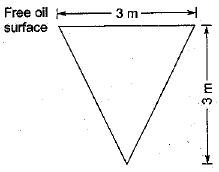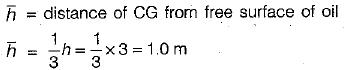Depth of pressure (h*) from free oil surface is given by,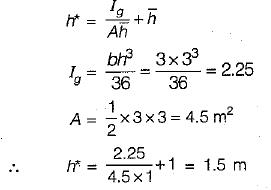QUESTION: 5

A vertically immersed surface of size b x d has its width b parallel to the water surface and depth of its centroid from the water surface is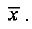The distance of its centre of water pressure from the water surface is

Solution:

Centre of water pressure,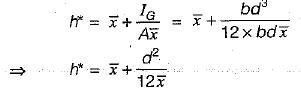QUESTION: 6

For a vertical semicircular plate submerged in a homogeneous liquid with its diameter ‘d' at the free surface, the depth of centre of pressure from the free surface is

Solution: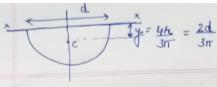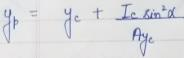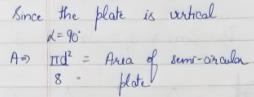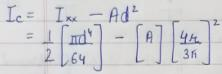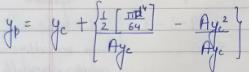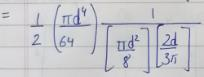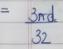QUESTION: 7

The position of centre of pressure on a plane surface immersed vertically in a static mass of fluid is

Solution:
QUESTION: 8

A vertical rectangular plane surface is submerged in water such that its top and bottom surfaces are 1.5 m and 6.0 m respectively below the free surface. The position of centre of pressure below the free surface will be at a distance of

Solution: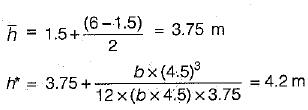QUESTION: 9

The horizontal component of force on a curved surface is equal to the

Solution:
QUESTION: 10

A circular plate of diameter d is submerged in water vertically, so that the topmost point is just at the water surface. The centre of pressure on the plate will be below the water surface at a depth of

Solution: